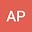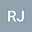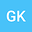The incomplete exponential \boldmath{$_pR_q(\alpha,\beta;z)$} function with some applications
•••• +1
• Ankit Pal,
• R. Jana,
• Ghazi Khammash,
• AJAY SHUKLA
Ankit Pal
Sardar Vallabhbhai National Institute of Technology
Author ProfileR. Jana
Sardar Vallabhbhai National Institute of Technology
Author ProfileGhazi Khammash
Al Aqsa University
Author ProfileAJAY SHUKLA
Sardar Vallabhbhai National Institute of Technology
Author Profile## Abstract

In this paper, we are motivated to establish a generalization of $_pR_q(\alpha,\beta;z)$ function in terms of incomplete exponential functions and obtain some properties of the incomplete exponential $_pR_q(\alpha,\beta;z)$ function. Further we give generating relations for incomplete exponential $_pR_q(\alpha,\beta;z)$ function. Some applications related to ground water pumping (Hydrology) modelling and probability theory have also been discussed.